# Financial forecasting with probabilistic programming and Pyro

Hi all again! Last year I have published several tutorials on financial forecasting using neural networks and I think some of the results were at least interesting and worth to apply in real trading applications. If you read them, you must have noticed, that when you try to fit some machine learning model on “random” data with hope to find hidden patterns you tend to overfit to the train set heavily. We used different regularization techniques and additional data to fight this problem, but it’s very time consuming and reminds blind search a bit.

Today I want to introduce a slightly different approach to fitting the same algorithms. Treating them with probabilistic point of view allows us to learn regularization from data per se, estimate certainty in our forecasts, use much less data for training and inject additional probabilistic dependencies in our models. I will not dive so much into technical or mathematical details of bayesian models or variational inference, I will give some overview, but also concentrate more on application. As always, you can check the code here.

I also recommend to check my previous tutorials on financial forecasting with neural nets:

For deeper understanding of probabilistic programming, Bayesian modeling and their applications, I recommend you to check following resources:

and to check out following Python libraries:

### Probabilistic programming

What is this probabilistic thing and why we call it programming? First of all, let’s remember what our “normal” neural nets are and what we get from them. We have parameters (weights), that are represented as matrices and outputs are normally some scalar values or vectors (in case of classification for instance). After we train this model with, let’s say, SGD, we have these matrices fixed and network supposed to output same vector on the same input sample. And this is totally correct! But what if we would’ve treated all these parameters and outputs as distributions depending on each other? Each weight in your neural network would be a sample from some distribution, the same as output — a sample from a whole net that depends on samples from parameters. What does it give to us?

Let’s start from basics. If we treat our network as a set of depending on each other distributions, this is first of all joint probability distribution p(y, z|x), where we have output y and some “inner”, latent variables of a model z depending on input x (all the same as with regular neural nets). We’re interested to find such neural net distributions, so we can sample y ~ p(y|x) and have our output as distribution (where expected value of samples from this distribution usually is the output, and standard deviation — estimate for uncertainty — the larger tails — the less we are confident about our output).

This setup is more or less clear, we just have to remember, that now all parameters, inputs and outputs in our model are distributions, and while training we need to fit parameters of these distributions to get better accuracy on real task. We also have to mention, that the shape of distributions of parameters we are setting by ourselves (for example, saying that all starting weights w ~ Normal(0, 1) and after we will learn correct mean and variance). Initial distributions are called priors and distributions with fit parameters after seeing training data — posteriors. The latter ones we use for sampling and getting the output.

How fitting of the model works? The general framework is called variational inference. Without getting into details we can assume, that we want to find such a model that maximized log likelihood p_w(z|x), where w are parameters of the model (parameters of distribution), z are our latent variables (hidden neurons outputs, that are sampled from distributions with parameters w) and x are input data samples. This is our model. In Pyro we introduce such a entity as guide for this model, that will consist simply of some distributions for all latent variables q_ф(z), where ф are called variational parameters. This distribution must approximate “real” distribution of the model parameters that fits data the best.

The training objective is to minimize expected value of [log(p_w(z|x)) — log(q_ф(z))] with respect to input data and samples from a guide. We will not discuss details of training process here, because it’s worth of several university courses and will do black box optimization for now.

Ah yes, why programming? Because we normally such probabilistic models (as neural nets) are described with directed graphs from one variable to other, and this way we show dependences of variables directly:

And originally such probabilistic programming languages were used to define such models and make inference on them.

#### Why probabilistic programming?

Instead of injecting dropouts or L1 regularization in your model, you can learn it from your data as additional latent variable. Taking into account that all weights are distributions, you can sample from them N times and get distribution of the output, where looking on the standard deviation you can estimate how confident your model is about the result. As a nice bonus, we need much less data for training such models and we are flexible on adding different dependencies between variables.

#### Why not probabilistic programming?

I don’t have yet huge experience with bayesian modeling, but what I have learnt from using Pyro and PyMC3, the training process is really long and it’s difficult to define correct prior distributions. Moreover, dealing with samples from distribution in production leads to misunderstandings and ambiguities.

### Data preparation

I have scrapped data for daily Ethereum prices from here. They include typical OHLCV (open high low close volume) tuples and, additionally, amount of daily tweets about Ethereum. We will use seven days of price, volume and tweet amount changes in % to predict next day percentage change.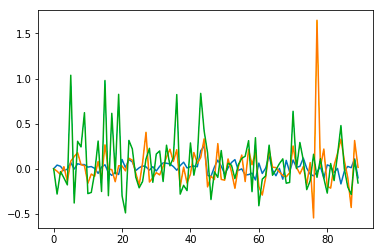prices, tweet amounts and volume changes

On the plot above you can see sample from data — blue is for price changes, yellow for tweet amount changes and green — for volume changes. There are some positive correlations between these values (0.1–0.2), so we expect to exploit some patters in data to train our model.

### Bayesian linear regression

First I wanted to check how simple linear regression will perform on our task (and I wanted to copy results from Pyro tutorial). We define our model in PyTorch following way (check more detailed explanations in official tutorial):

`class RegressionModel(nn.Module):    def __init__(self, p):        super(RegressionModel, self).__init__()        self.linear = nn.Linear(p, 1)`
`def forward(self, x):        # x * w + b        return self.linear(x)`

But this is simple deterministic model as we used to work with, but this is the way to define probabilistic one in Pyro:

`def model(data):    # Create unit normal priors over the parameters    mu = Variable(torch.zeros(1, p)).type_as(data)    sigma = Variable(torch.ones(1, p)).type_as(data)    bias_mu = Variable(torch.zeros(1)).type_as(data)    bias_sigma = Variable(torch.ones(1)).type_as(data)    w_prior, b_prior = Normal(mu, sigma), Normal(bias_mu, bias_sigma)    priors = {'linear.weight': w_prior, 'linear.bias': b_prior}    lifted_module = pyro.random_module("module", regression_model, priors)    lifted_reg_model = lifted_module()`
`with pyro.iarange("map", N, subsample=data):        x_data = data[:, :-1]        y_data = data[:, -1]        # run the regressor forward conditioned on inputs        prediction_mean = lifted_reg_model(x_data).squeeze()        pyro.sample("obs",                    Normal(prediction_mean, Variable(torch.ones(data.size(0))).type_as(data)),                    obs=y_data.squeeze())`

In the code above you can see, that we set for out general linear regression model distributions for parameters W and b, and both are ~Normal(0, 1). We call them priors, create Pyro’s random function (RegressionModel in PyTorch in our case), add priors to it ({‘linear.weight’: w_prior, ‘linear.bias’: b_prior}) and sample from this model p(y|x) based on input data x.

And the guide for the model will look like this:

`def guide(data):    w_mu = Variable(torch.randn(1, p).type_as(data.data), requires_grad=True)    w_log_sig = Variable(0.1 * torch.ones(1, p).type_as(data.data), requires_grad=True)    b_mu = Variable(torch.randn(1).type_as(data.data), requires_grad=True)    b_log_sig = Variable(0.1 * torch.ones(1).type_as(data.data), requires_grad=True)    mw_param = pyro.param("guide_mean_weight", w_mu)    sw_param = softplus(pyro.param("guide_log_sigma_weight", w_log_sig))    mb_param = pyro.param("guide_mean_bias", b_mu)    sb_param = softplus(pyro.param("guide_log_sigma_bias", b_log_sig))    w_dist = Normal(mw_param, sw_param)    b_dist = Normal(mb_param, sb_param)    dists = {'linear.weight': w_dist, 'linear.bias': b_dist}    lifted_module = pyro.random_module("module", regression_model, dists)    return lifted_module()`

Here we define the variational distribution for distributions we want to “train”. As you can see, we define same shape distributions for W and b, but make them more close to reality (as far as we can assume). In this example I make them a bit more narrow (~Normal(0, 0.1)).

After we train model this way:

`for j in range(3000):    epoch_loss = 0.0    perm = torch.randperm(N)    # shuffle data    data = data[perm]    # get indices of each batch    all_batches = get_batch_indices(N, 64)    for ix, batch_start in enumerate(all_batches[:-1]):        batch_end = all_batches[ix + 1]        batch_data = data[batch_start: batch_end]        epoch_loss += svi.step(batch_data)`

After fitting we want to sample y from the model. We do it 100 times and we will check means and standard deviations for each time step prediction (and as higher will be standard deviation, then less confident we can be about this prediction).

`preds = []for i in range(100):    sampled_reg_model = guide(X_test)    pred = sampled_reg_model(X_test).data.numpy().flatten()    preds.append(pred)`

As we can remember, with financial forecasts classic metrics as MSE, MAE or MAPE can be a bit confusing — relatively small error rates don’t mean that your model works well, it’s always important to check performance visually on out-of-sample data, and that’s what we do: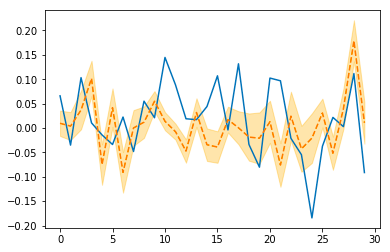bayesian model forecasts for 30 days

As we can see, it doesn’t work really good, but nice forecast shape in the last jump gives us small hope. Let’s move forward!

### Regular neural network

After this very simple model we want to try something much more interesting as neural network. First let’s learn a simple MLP with single hidden layer containing 25 neurons with linear activations:

`def get_model(input_size):    main_input = Input(shape=(input_size, ), name='main_input')    x = Dense(25, activation='linear')(main_input)    output = Dense(1, activation = "linear", name = "out")(x)    final_model = Model(inputs=[main_input], outputs=[output])    final_model.compile(optimizer='adam',  loss='mse')    return final_model`

And train it for 100 epochs:

`model = get_model(len(X_train))history = model.fit(X_train, Y_train,               epochs = 100,               batch_size = 64,               verbose=1,               validation_data=(X_test, Y_test),              callbacks=[reduce_lr, checkpointer],              shuffle=True)`

And get following results: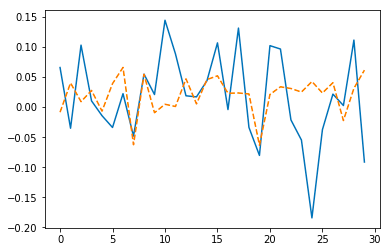Keras neural network forecasts for 30 days

I think it’s even worse than simple bayesian regression, moreover this model can’t get certainty estimates and what’s more important, this model even isn’t regularized.

### Bayesian neural network

Now I want to define the same neural network we trained in Keras, but in PyTorch:

`class Net(torch.nn.Module):    def __init__(self, n_feature, n_hidden):        super(Net, self).__init__()        self.hidden = torch.nn.Linear(n_feature, n_hidden)   # hidden layer        self.predict = torch.nn.Linear(n_hidden, 1)   # output layer`
`def forward(self, x):        x = self.hidden(x)        x = self.predict(x)        return x`

Comparing to bayesian regression model we have two sets of parameters now (from input to hidden layer and from hidden layer to the output), so we change slightly distribution and priors for our model:

`priors = {'hidden.weight': w_prior,               'hidden.bias': b_prior,              'predict.weight': w_prior2,              'predict.bias': b_prior2}`

and the guide:

`dists = {'hidden.weight': w_dist,               'hidden.bias': b_dist,              'predict.weight': w_dist2,              'predict.bias': b_dist2}`

Don’t forget to set different names for all distributions in the models, because there shouldn’t be any ambiguity and repetitions! Check more details in the source code. Let’s check final results after fitting the model and sampling: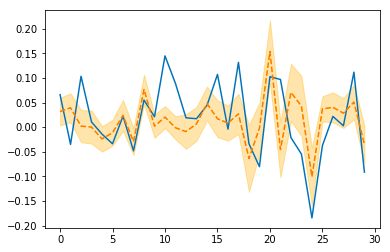Pyro neural network forecasts for 30 days

It looks much-much better than any of previous results!

Concerning regularization or nature of the weights learnt by Bayesian model comparing to the regular one, I would like also to see statistics of the weights. This is how I check parameters of Pyro model:

`for name in pyro.get_param_store().get_all_param_names():    print name, pyro.param(name).data.numpy()`

And this is how I do this with Keras model:

`import tensorflow as tfsess = tf.Session()with sess.as_default():    tf.global_variables_initializer().run()`
`dense_weights, out_weights = None, Nonewith sess.as_default():    for layer in model.layers:        if len(layer.weights) > 0:            weights = layer.get_weights()            if 'dense' in layer.name:                dense_weights = layer.weights.eval()            if 'out' in layer.name:                out_weights = layer.weights.eval()`

For example, for Keras model last layer’s weights have mean and standard deviation -0.0025901748, 0.30395043 and Pyro model has them equal to 0.0005974418, 0.0005974418. A lot smaller and this is good! That’s what a lot of regularizations like L2 or Dropout do — push our parameters to zero and we could achieve it with variational inference! For hidden layer weights situation is even more interesting. Let’s plot some weight vectors as graphs, blue are weights of Keras, and orange are weights for Pyro: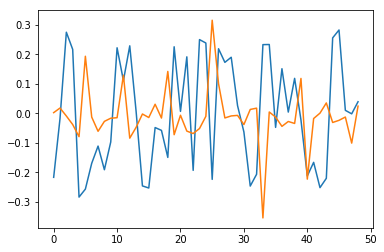some weights between input and hidden layer

What is really interesting, that’s the fact that not just mean and standard deviation of weights is smaller, moreover, weights became sparse, so basically we learnt a sparse representation (kind of L1) for first set of weights and some kind of L2 regularization for second, which is amazing! Don’t forget to launch the code!

### Conclusion

We used novel approach for training neural nets. Instead of sequential updating static weights we were updating distribution of weights, and, so, we could achieve interesting and promising results. I want to underline, that bayesian methods gave us opportunity to regularize neural nets without adding regularizer manually, understand uncertainty of models, and, potentially, using less data for better results. Stay tuned! :)

P.S.
Follow me also in Facebook for AI articles that are too short for Medium, Instagram for personal stuff and Linkedin!